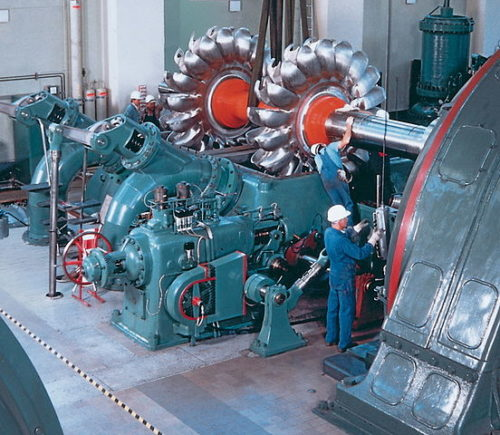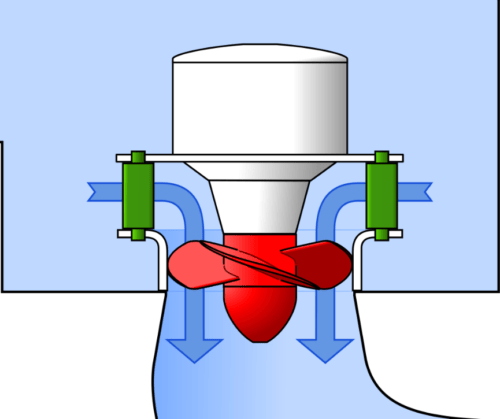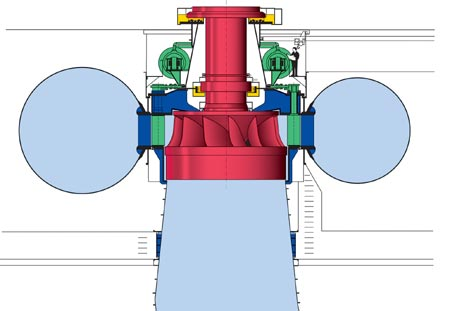# Turbine | Types, Working [Fully Explained with Images]

By | October 1, 2018

## What is a turbine?

Before moving to the definition of turbine and how it works, let’s first understand the meaning of the word from which turbine has derived its name.

The word turbine has been formed from the Latin word ‘Turbo’, which means ‘to spin’.

Now you can easily relate the meaning of the word to the turbines which rotates about its axis.

Definition of turbine

A turbine is a Mechanical device which is used to convert energy of moving fluid into the Mechanical energy.

This mechanical energy can further be used to either generate electricity or to perform any other useful work, like machining, milling etc.

## Working of turbines

All the turbines convert the energy of the moving fluid into useful mechanical work with the help of their blades. These blades provide resistance to the flow of fluid and hence convert their kinetic energy into the rotational movement of turbine shaft. As a consequence, moving fluid loses its kinetic energy.

## Types of turbines

Turbines can be broadly classified into two types based on the method of energy transfer.

1. ### Impulse turbine

In impulse turbine the transfer of power between the working fluid and the turbine happens instantly. In a single step all the kinetic energy of fluid gets converted into mechanical energy of turbine.

Pelton turbine is an example of impulse turbine.Pelton turbine

1. ### Reaction Turbine

In reaction turbine the kinetic energy of the working fluid gets converted into the mechanical energy of the turbine in some progressive steps (which is known as reaction).

Kaplan and Francis turbines are the examples of reaction turbines.

Reaction turbines can further be classified into three types. These types are based on the inlet of working fluid into the turbine.

• #### Axial flow turbine

In axial flow turbine the working fluid enters parallel to the axis of rotation of turbine.

Kaplan turbine is an example of axial flow turbine. Below is the diagram of Kaplan Turbine.Kaplan Turbine

• #### Radial flow turbine

In radial flow turbine the working fluid enters tangentially to the axis of rotation of turbine.

Francis turbine is an example of Radial flow turbine.

Below is the diagram of Francis turbine.Francis turbine

• #### Mixed flow turbine

These turbines use combines flow i.e. radial and axial. These turbines are most used now a day.

Turbines are mainly used for power generation purpose now a day. An electrical alternator is used to generate electricity from the rotary motion of the turbine shaft. These turbines can work with Air, Gas, Steam, Water etc. as a working fluid.

Image sources:

Pelton turbine: Attribution: Von Voith Siemens Hydro Power – http://www.voithsiemens.com, CC BY-SA 3.0, https://commons.wikimedia.org/w/index.php?curid=544820

Kaplan turbine: Attribution: By Jahobr – Own work, CC0, https://commons.wikimedia.org/w/index.php?curid=57640260

Francis turbine: Attribution: CC BY-SA 3.0, https://commons.wikimedia.org/w/index.php?curid=463246

This site uses Akismet to reduce spam. Learn how your comment data is processed.